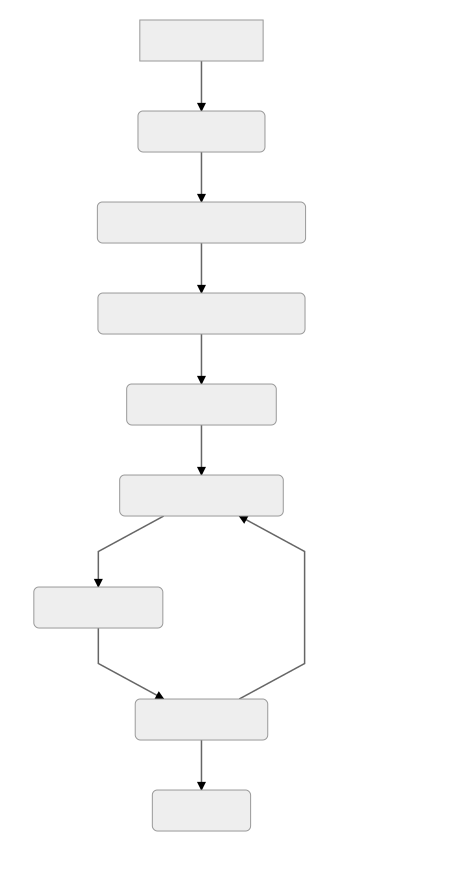Back to the Main Page

# Tips for supervisions

I recommend that supervisors get students to bring their laptops to the supervisions or, if that is not possible, ideally the supervisor can bring a laptop.

A probably sheet is provide, which contains problems similar in style to the question that will be on the exam. These will have to be answered with pen and paper in the exam; however, it might be helpful for students to try write and run the code in `Matlab` during the supervisions.

All the code requires only the base `Matlab` installation. If it's not possible to install `Matlab` on the student's or supervisor's computer then it will be possible to run the code using Matlab Online. To access `Matlab Online` it is necessary to have a Mathworks account (there is Create Account link on the `Matlab Online` page). They will also need to associate their `Matlab` license code with their Mathworks Account. A free `Matlab` license code can be obtained from the UIS Software Distribution site.

## Answers to the supervision problems

### Problem 1a

The function runs correctly in all cases. In the first case, `A = 'small'`, because `'small'` is assigned to the output variable (`output1`) when the input (`'input1'`) is equal to `1`. In the second case, `B = 'big'`, because `'big'` is assigned to the output variable when the input variable is any value other than `1`. That is, `'big'` is assigned to the output variable in all cases where `input1` being equal to `1` is not met.

### Problem 1b

The function would run correctly when the input is equal to `1` and when it is equal to `'one'`. It would produce an error when the input is equal to `-10` or any value other than `1` or `one`. When the input is equal to any other value then neither the first condition is met nor the second condition and therefore no value is assigned to the variable `output1`. As a result the function can't produce an output and so it produces and error. When the input is `1`, the output is `'small'`, and when the input is `'one'` the output is `'big'`.

### Problem 2a

There are many different solutions to this problem. A couple of approaches are shown.

Example 1

``````function CountFun(x) % declare function
for y = x
switch y
case 1
disp('one')
case 2
disp('two')
case 3
disp('three')
end
end``````

Example 2

``````function CountFun(x)

arrayfun(@(y) NumberToWord(y),x)

function NumberToWord(y)
switch y
case 1
disp('one')
case 2
disp('two')
case 3
disp('three')
end``````

Example 3

``````function CountFun(x)

for i = 1 : length(x)
y = x(i);
if y == 1
disp('one')
elseif y ==  2
disp('two')
elseif y ==  3
disp('three')
end
end``````

### Problem 2b

The solution to problem 2b is of the same general format as problem 2a. However, if using an series of `if` or `if` and `elseif` statements then `strcmp()` must be used in place of `==`